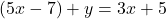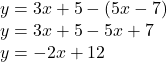## if the sum of two expressions is 3x+5 and one expression is 5x-7 what is the other expression

Question

if the sum of two expressions is 3x+5 and one expression is 5x-7 what is the other expression

in progress 0
3 months 2021-08-28T08:18:24+00:00 1 Answers 2 views 0

-2x + 12

Step-by-step explanation:

If we call our other expression y, we can set up an equation tying together 3x + 5, y, and 5x – 7:Solving for y by subtracting 5x – 7 from both sides will get us the expression we’re looking for:So the other expression in the sum is -2x + 12.